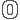NMEA 0183

NMEA is a standard protocol, used by GPS receivers to transmit data. NMEA output is EIA-422A but for most purposes you can consider it is RS-232 compatible. Use 4800 bps, 8 data bits, no parity and one stop bit (8N1). NMEA 0183 sentences are all ASCII. Each sentence begins with a dollarsign (\$) and ends with a carriage return linefeed (<CR><LF>). Data is comma delimited. All commas must be included as they act as markers. Some GPS do not send some of the fields. A checksum is optionally added (in a few cases it is mandatory). Following the \$ is the address field aaccc. aa is the device id. GP is used to identify GPS data. Transmission of the device ID is usually optional. ccc is the sentence formatter, otherwise known as the sentence name.RMB
```\$GPRMB,A,x.x,a,c--c,d--d,llll.ll,e,yyyyy.yy,f,g.g,h.h,i.i,j*kk

RMB  = Recommended Minimum Navigation Information

2    = Crosstrack error in nautical miles

3    = Direction to steer (L or R) to correct error

4    = Origin waypoint ID#

5    = Destination waypoint ID#

6    = Destination waypoint latitude

7    = N or S

8    = Destination waypoint longitude

9    = E or W

10   = Range to destination in nautical miles

11   = Bearing to destination, degrees True

12   = Destination closing velocity in knots

13   = Arrival status; (A=entered or perpendicular passed)

14   = Checksum```RMC
```\$GPRMC,hhmmss.ss,A,llll.ll,a,yyyyy.yy,a,x.x,x.x,ddmmyy,x.x,a*hh

RMC  = Recommended Minimum Specific GPS/TRANSIT Data

1    = UTC of position fix

3    = Latitude of fix

4    = N or S

5    = Longitude of fix

6    = E or W

7    = Speed over ground in knots

8    = Track made good in degrees True

9    = UT date

10   = Magnetic variation degrees (Easterly var. subtracts from true course)

11   = E or W

12   = Checksum```GGA
```\$GPGGA,hhmmss.ss,llll.ll,a,yyyyy.yy,a,x,xx,x.x,x.x,M,x.x,M,x.x,xxxx*hh

GGA  = Global Positioning System Fix Data

1    = UTC of Position

2    = Latitude

3    = N or S

4    = Longitude

5    = E or W

6    = GPS quality indicator (0=invalid; 1=GPS fix; 2=Diff. GPS fix)

7    = Number of satellites in use [not those in view]

8    = Horizontal dilution of position

9    = Antenna altitude above/below mean sea level (geoid)

10   = Meters  (Antenna height unit)

11   = Geoidal separation (Diff. between WGS-84 earth ellipsoid and
mean sea level.  -=geoid is below WGS-84 ellipsoid)
12   = Meters  (Units of geoidal separation)

13   = Age in seconds since last update from diff. reference station

14   = Diff. reference station ID#

15   = Checksum```VTG
```\$GPVTG,t,T,,,s.ss,N,s.ss,K*hh

VTG  = Actual track made good and speed over ground

2    = Fixed text 'T' indicates that track made good is relative to true north

3    = not used

4    = not used

5    = Speed over ground in knots

6    = Fixed text 'N' indicates that speed over ground in in knots

7    = Speed over ground in kilometers/hour

8    = Fixed text 'K' indicates that speed over ground is in kilometers/hour

9    = Checksum```RMA
```\$GPRMA,A,llll.ll,N,lllll.ll,W,,,ss.s,ccc,vv.v,W*hh

RMA  = Navigation data from present position

1    = Data status

2    = Latitude

3    = N/S

4    = longitude

5    = W/E

6    = not used

7    = not used

8    = Speed over ground in knots

9    = Course over ground

10   = Variation

11   = Direction of variation E/W

12   = Checksum```GSA
```\$GPGSA,A,3,19,28,14,18,27,22,31,39,,,,,1.7,1.0,1.3*35

GSA  = GPS receiver operating mode, SVs used for navigation, and DOP values.

1    = Mode:

M=Manual, forced to operate in 2D or 3D

A=Automatic, 3D/2D

2    = Mode:

1=Fix not available

2=2D

3=3D

3-14 = IDs of SVs used in position fix (null for unused fields)

15   = PDOP

16   = HDOP

17   = VDOP```GSV
```\$GPGSV,4,1,13,02,02,213,,03,-3,000,,11,00,121,,14,13,172,05*67

GSV  = Number of SVs in view, PRN numbers, elevation, azimuth & SNR values.

1    = Total number of messages of this type in this cycle

2    = Message number

3    = Total number of SVs in view

4    = SV PRN number

5    = Elevation in degrees, 90 maximum

6    = Azimuth, degrees from true north, 000 to 359

7    = SNR, 00-99 dB (null when not tracking)

8-11 = Information about second SV, same as field 4-7

12-15= Information about third SV, same as field 4-7

16-19= Information about fourth SV, same as field 4-7

```